# Part 6 : Following along MIT intro to deep learningAbhijit Ramesh / March 31, 2021

11 min read––– views

## Deep Learning and expressivity of Neural Nets

#### Universal Approximation Therom

This theorem states that, A feedforward network with a single layer is sufficient to approximate, to an arbitrary precision, any continuous function.

We have seen many neural networks in the past and they have been called Deep Neural Networks because of the fast that it works on the principle of stacking neural networks on top of each other but this theorem states the opposite and says that for every problem that can be framed into something that when given an input should give an output there exist a neural net with a single layer that could solve this problem

There are some issues with this claim,

1. There is no mention of the number of hidden units that are required for the same, it could be an infeasibly large number.
2. This does not claim that the model may generalise on the problem it just says that a model should fit for the problem.

The problem with any technology is that understanding it to a level that is not complete makes it overhyped. We might end up marketing the technology in such a way that it accidentally conveys the message that it could be used in solving some problem that cannot be or may not be the most feasible for the use-case. By saying fully understanding a problem it does not mean that we should know every single aspect of it and should be able to say any theory based on that technology from thin air, it means that we should know the limitations of the technology as well.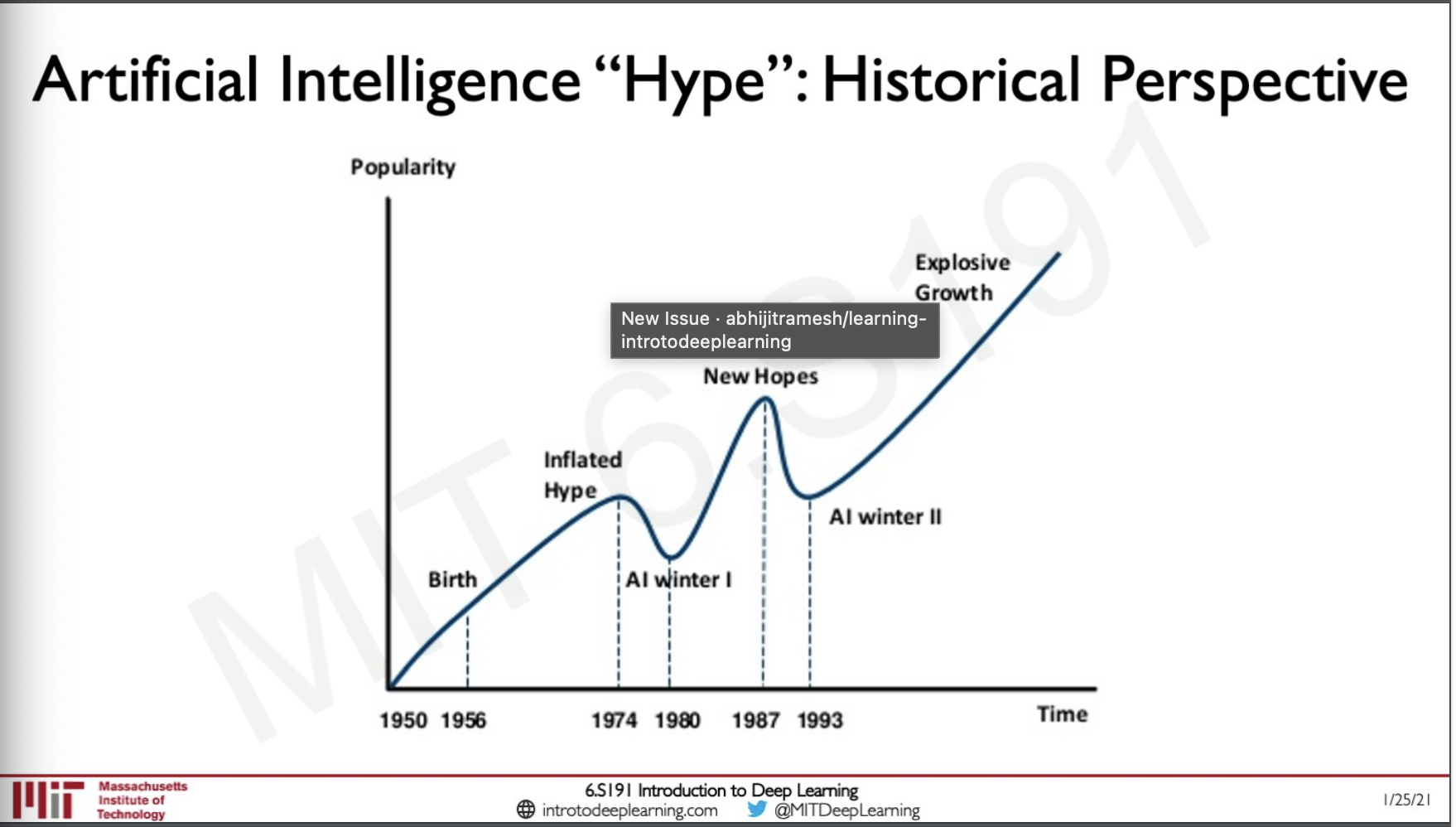In the past there had been two AI winters, what this means is that research and development in the field of AI have stopped because of the limitations of resources or could even be the case that we were trying to solve a dead-end problem, But in recent years from 1993 there has been an explosive growth in R&D on AI and we have been seeing and using many applications that leverage AI for many years now. TL;DR what this means is that we should understand the limitations of AI and also know what are some of the frontiers in AI that has good scope for research.

## Limitations

Understanding Deep Neural Networks Requires Rethinking Generalisation - is a very famous paper on deep learning, In brief, what they did was to take some images and then flip a k sided die randomly for these images, (k for each of the class labels) depending upon the flip of the die a label is then assigned to the image.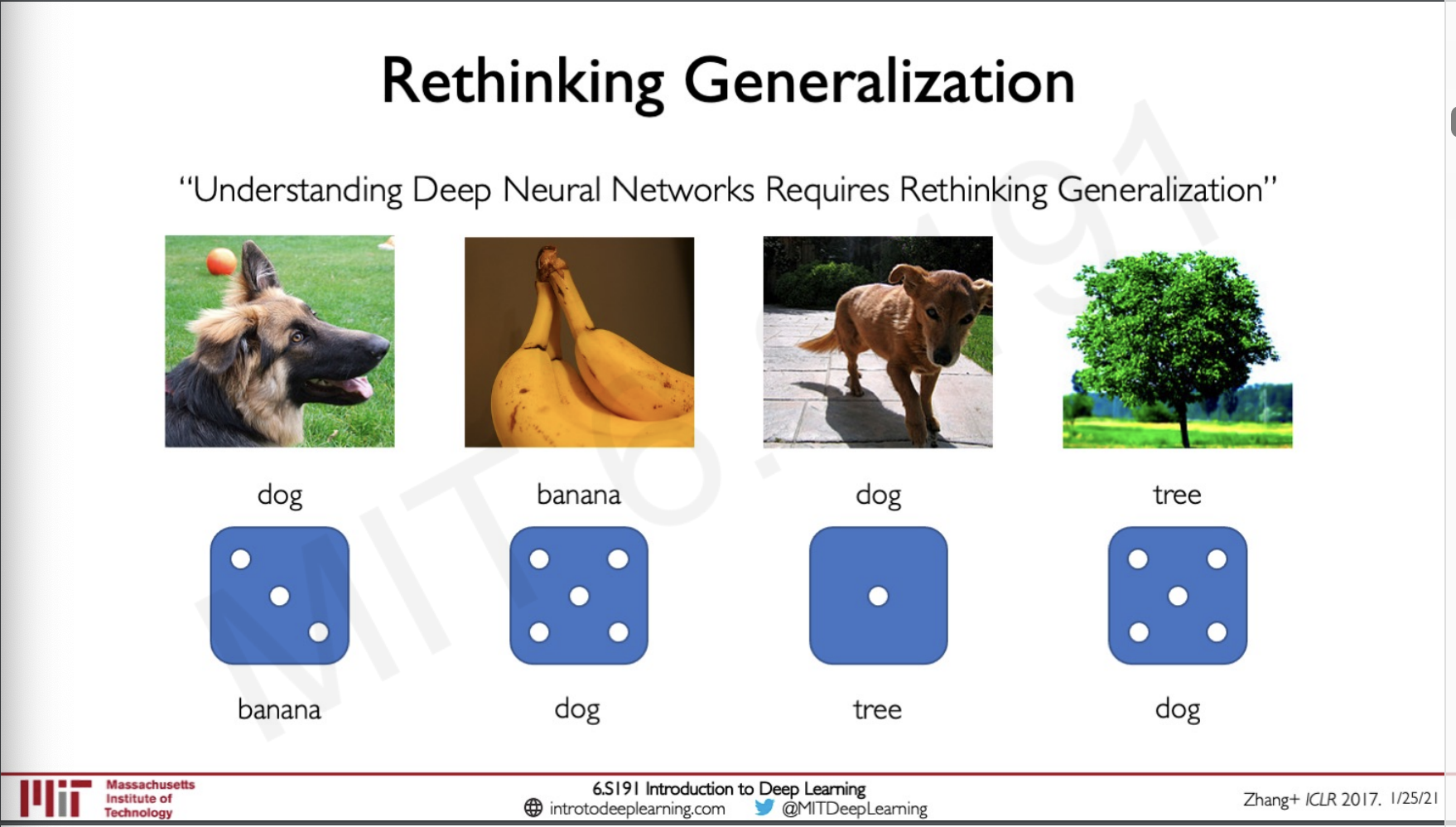So depending on this means that some of the images in the same class would have very different labels as seen from above for the case of the dog class.

After this the neural network was trained for the same and the as the randomness keep on increasing the model performed worse in test set, but for the training set given enough time the neural network would start showing an accuracy of 100%.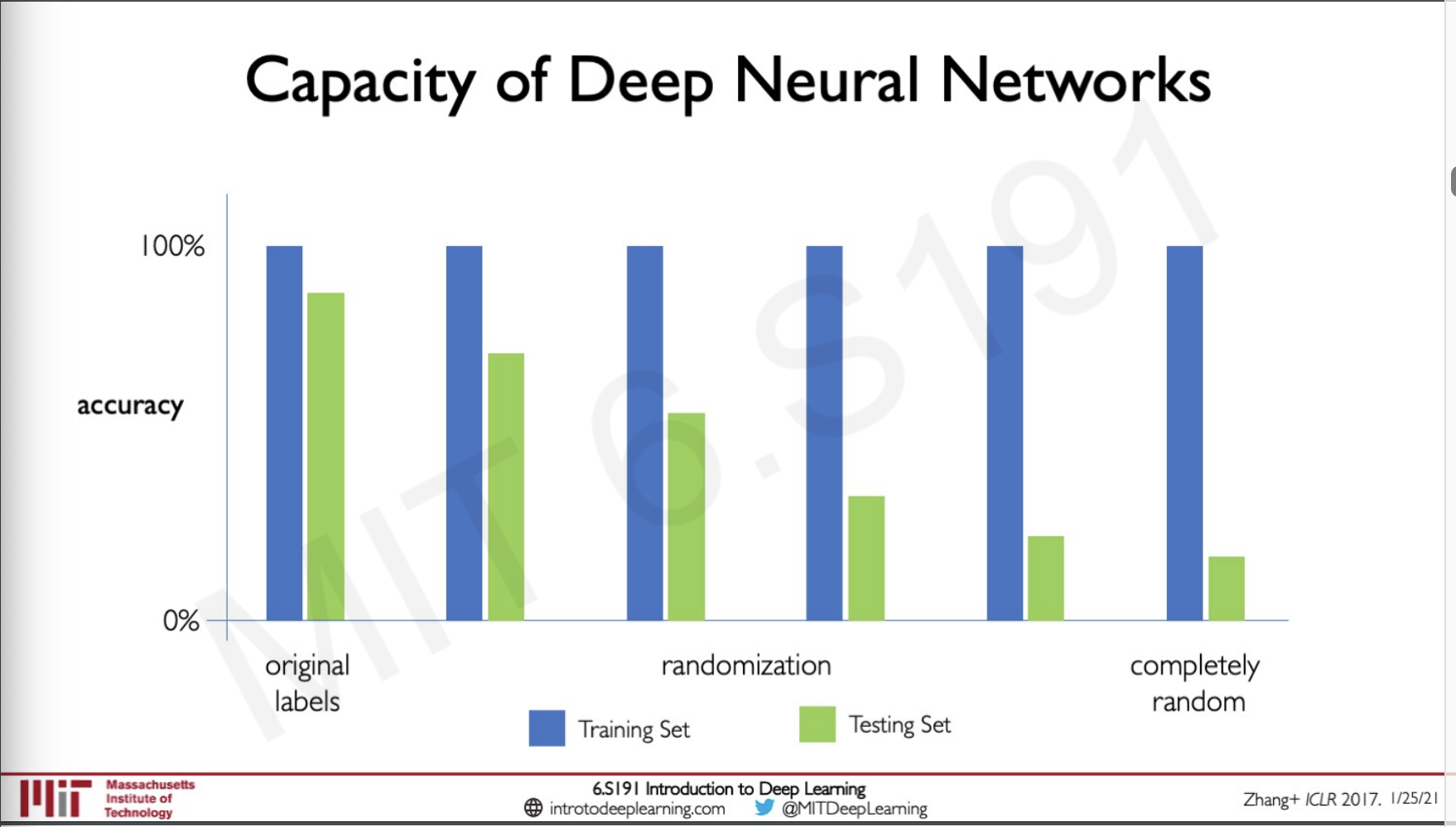So here the neural network is actually fitting to completely random data.

What this proves is that neural networks are excellent function approximations, so lets say we have a network that is trained on some data and we get the following graph as the function,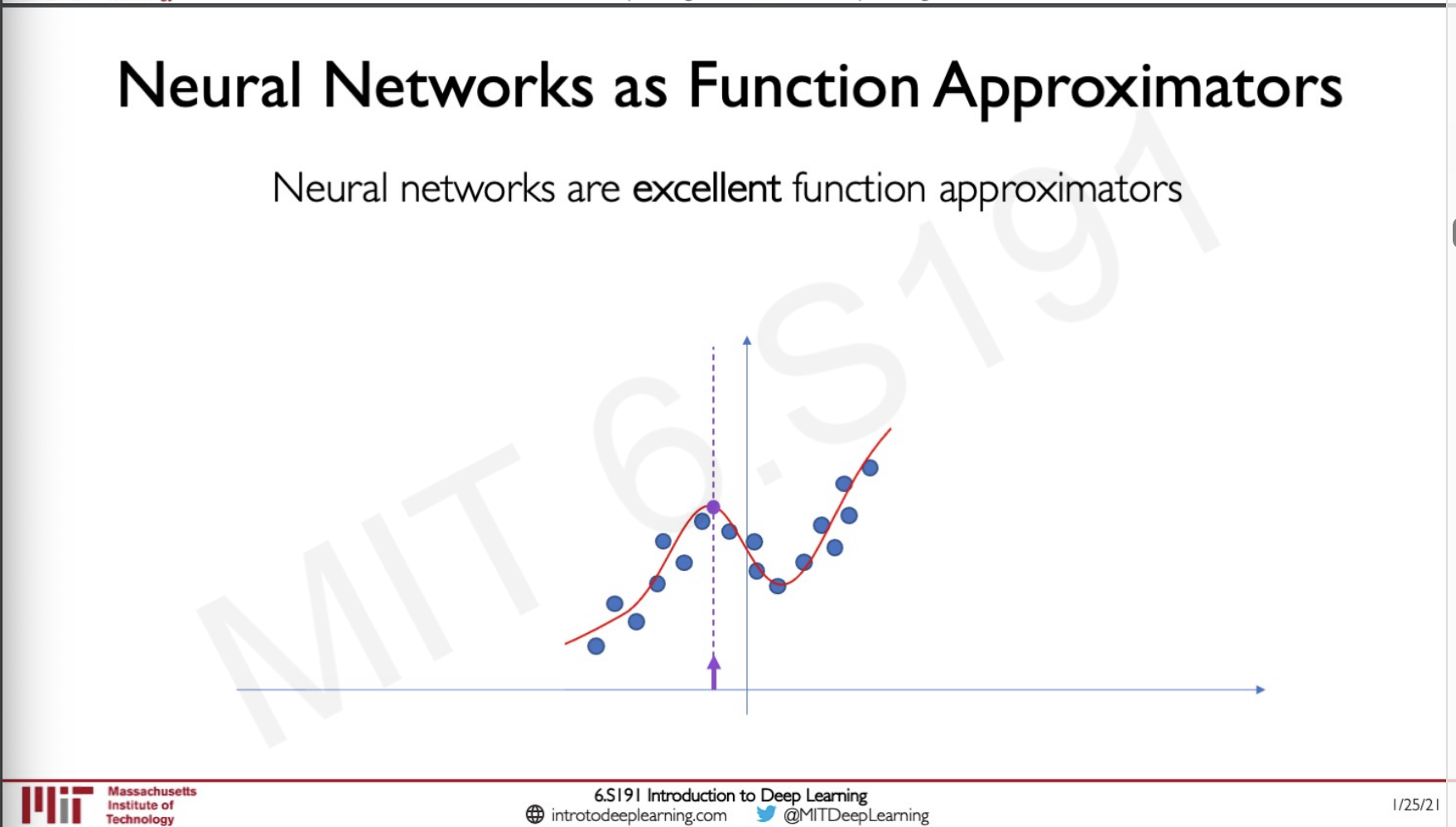given any point (purple) the Neural Net is likely to predict the maximum likely hood of where that point would lie on the function.

But lets say that now we have extend the limit of this function beyond the limit of the data which it has been trained on.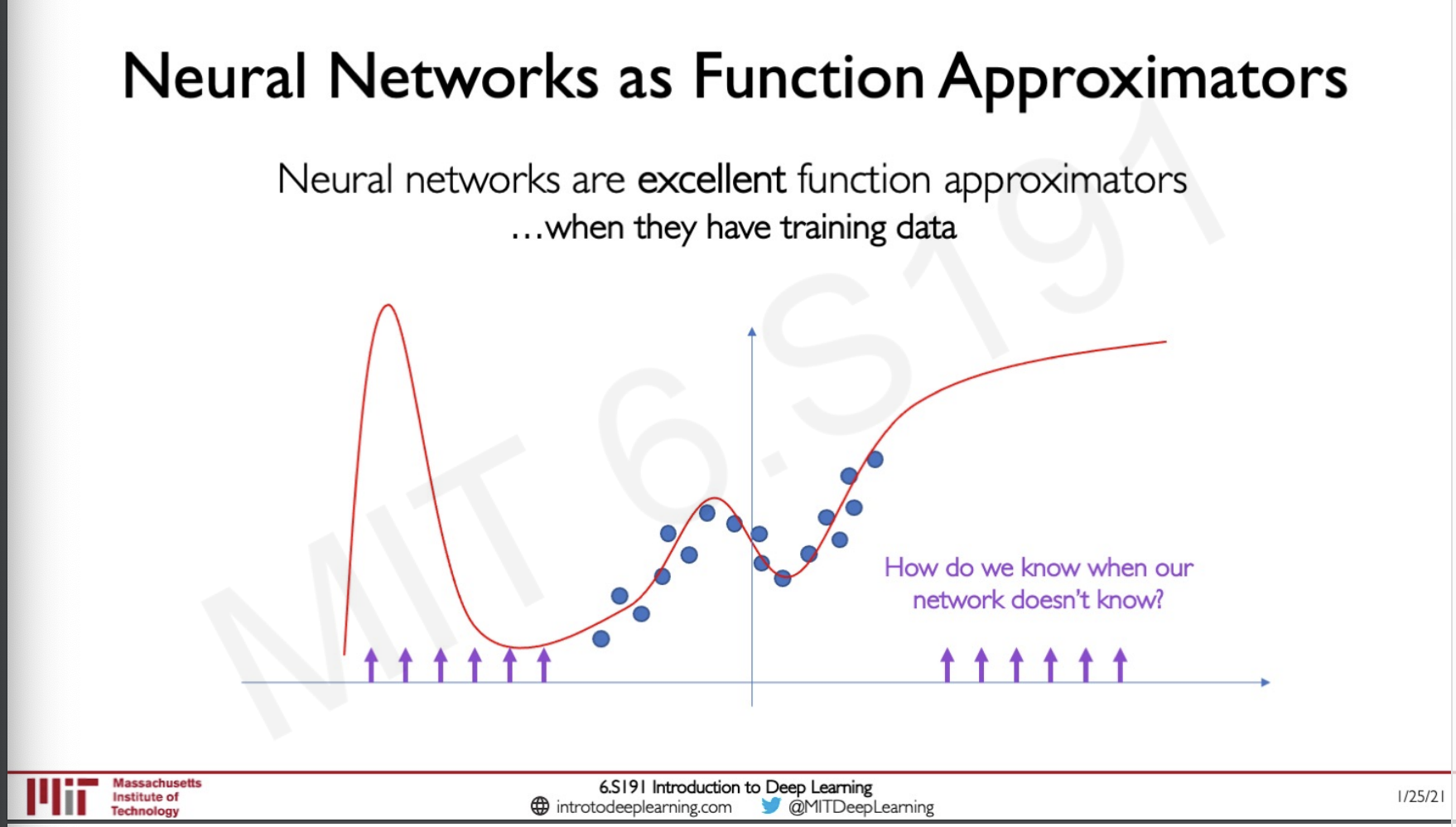Now the neural net does not know where to place the point given an input because it does not have any trained data, this actually suggest a change to the Universal Approximation Theorem, Neural networks are excellent function approximators when they have training data.

## Neural Network Faliour Modes

People view machine learning as alchemy, one magic solution to all problems. There is also a misconception that if we have some random data, we can select some random network architecture tweak some learning parameters and get excellent results. This is not true at all, or the model is going to be only as good as our data.

Let us take an example where a neural network was provided with the following data.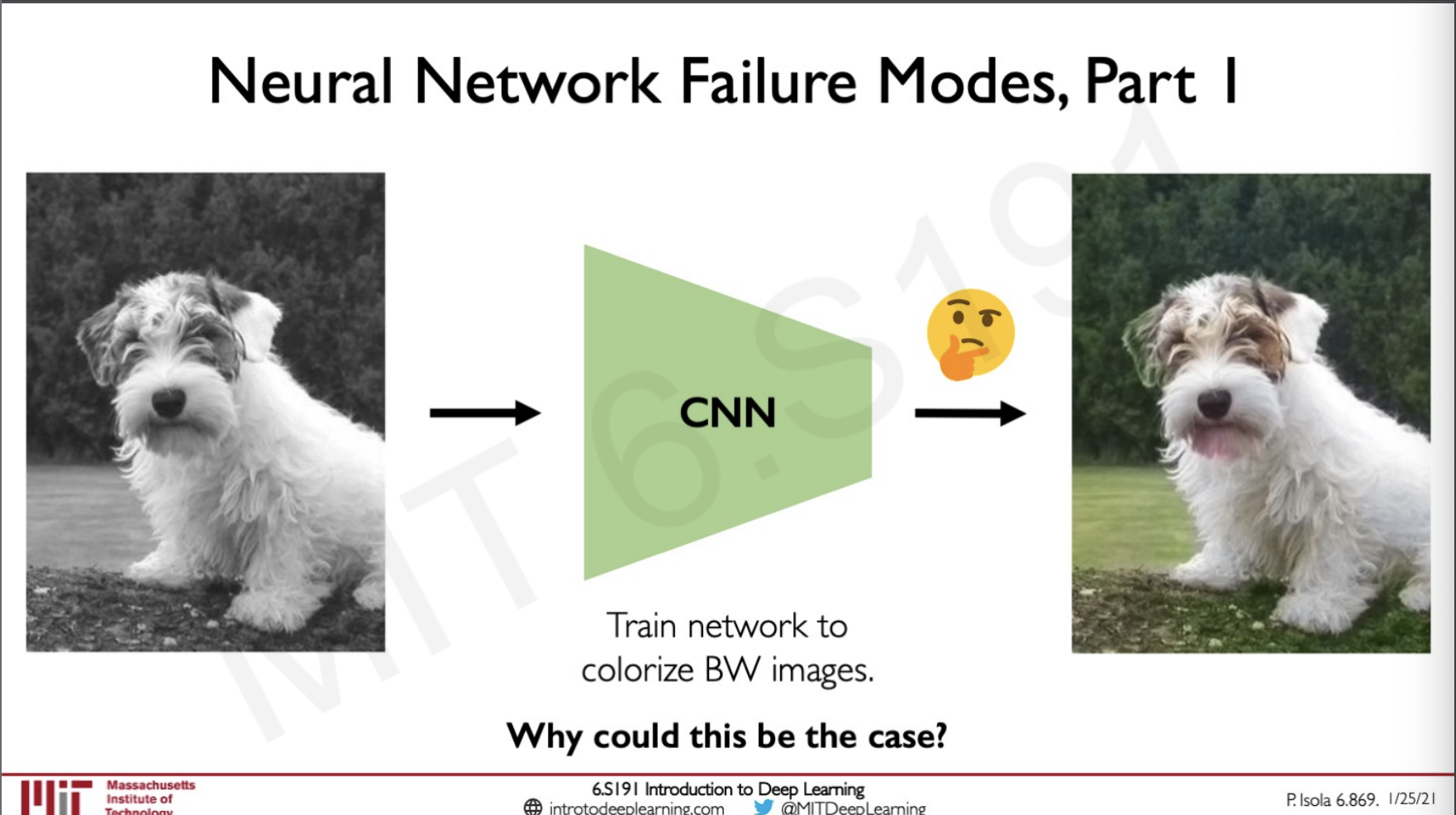Here, the network transfers black and white images to colour images, but there is a pinkish hue under the dog's nose even though its mouth appears closed in the black and white image. What could be the reason for this?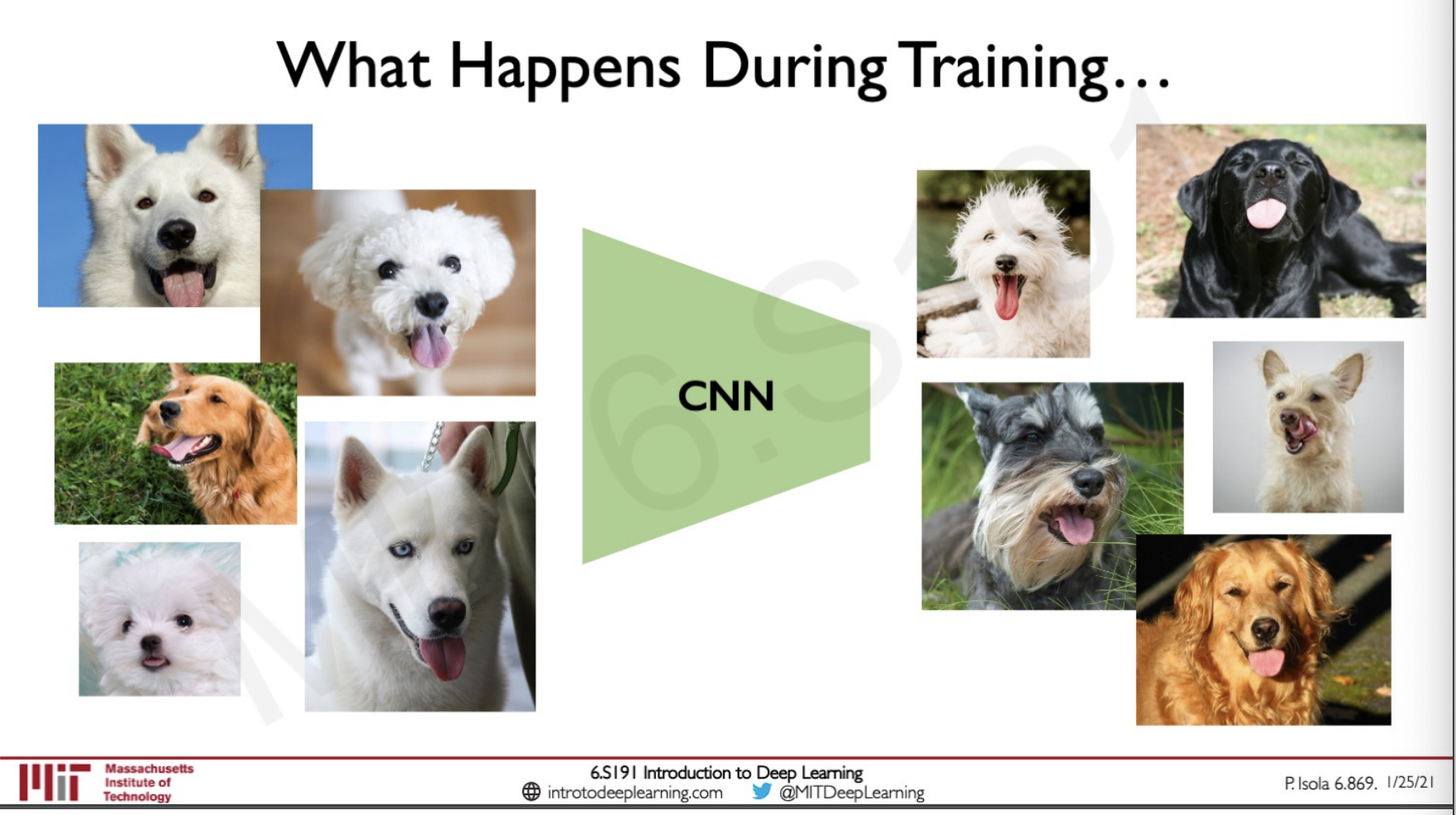The image might have been trained over this dataset, where most of the dogs have there tongue out so the model is trained on this and hence the result has the pinkish hue.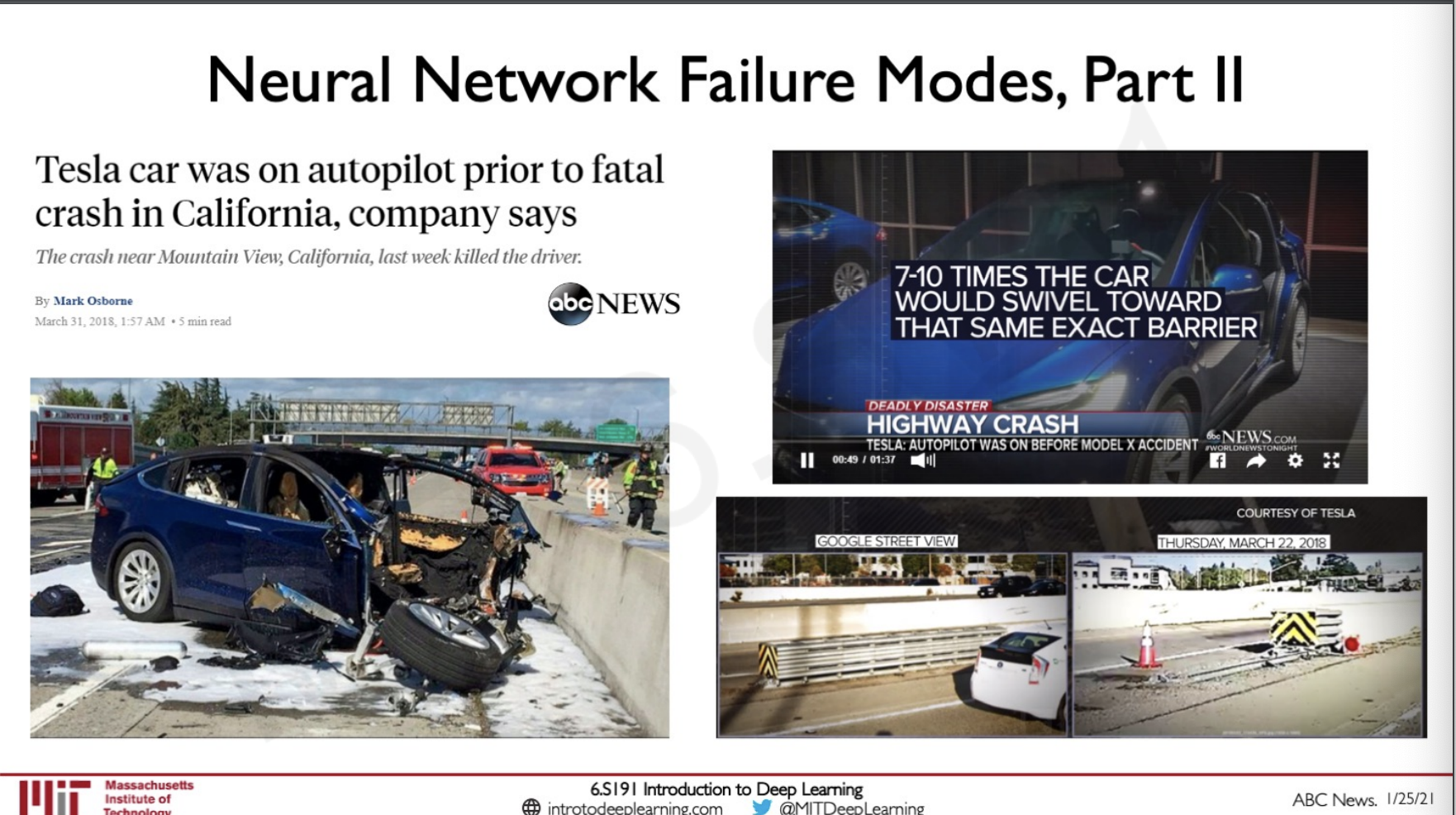On March 31st of 2018, there has been an incident where a tesla car crashed into a Barrie while using autopilot, causing the driver's tragic death. It was also said that the driver has reported multiple times that the car was swivelling towards the barrier before when this event was investigated the data that was supposed to be representative for governing the driving of the car was not updated with new construction that happened in the road leading to the neural network not being able to predict what is to be done when it encountered the new barrier and thus caused in the crash of the car.

The message here is the your model is only going to be as good as your data.

## Uncertanity in Deep Learning

When should we really be concerned about a neural network giving a wrong prediction ?

The more the AI is becoming closer to over lives we should be more concerned about how likely the model is to predict if something incorrectly.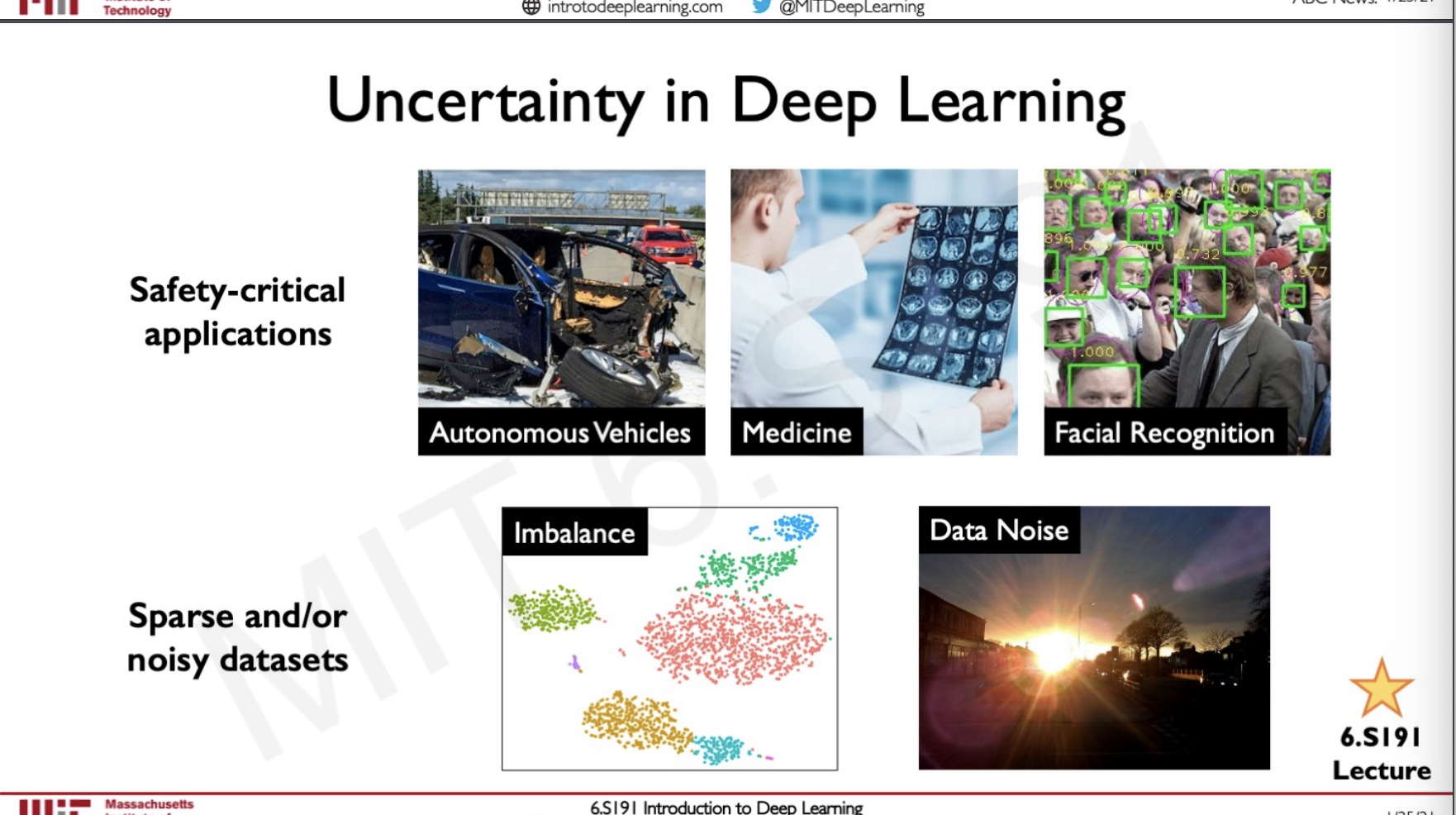Let us say we have a model that predicts if a given image is a cat or a dog and the prediction is given as a probability.

When giving an output the probabilities should sum upto 1

So what if in the training data these is a model which has both cat and a dog.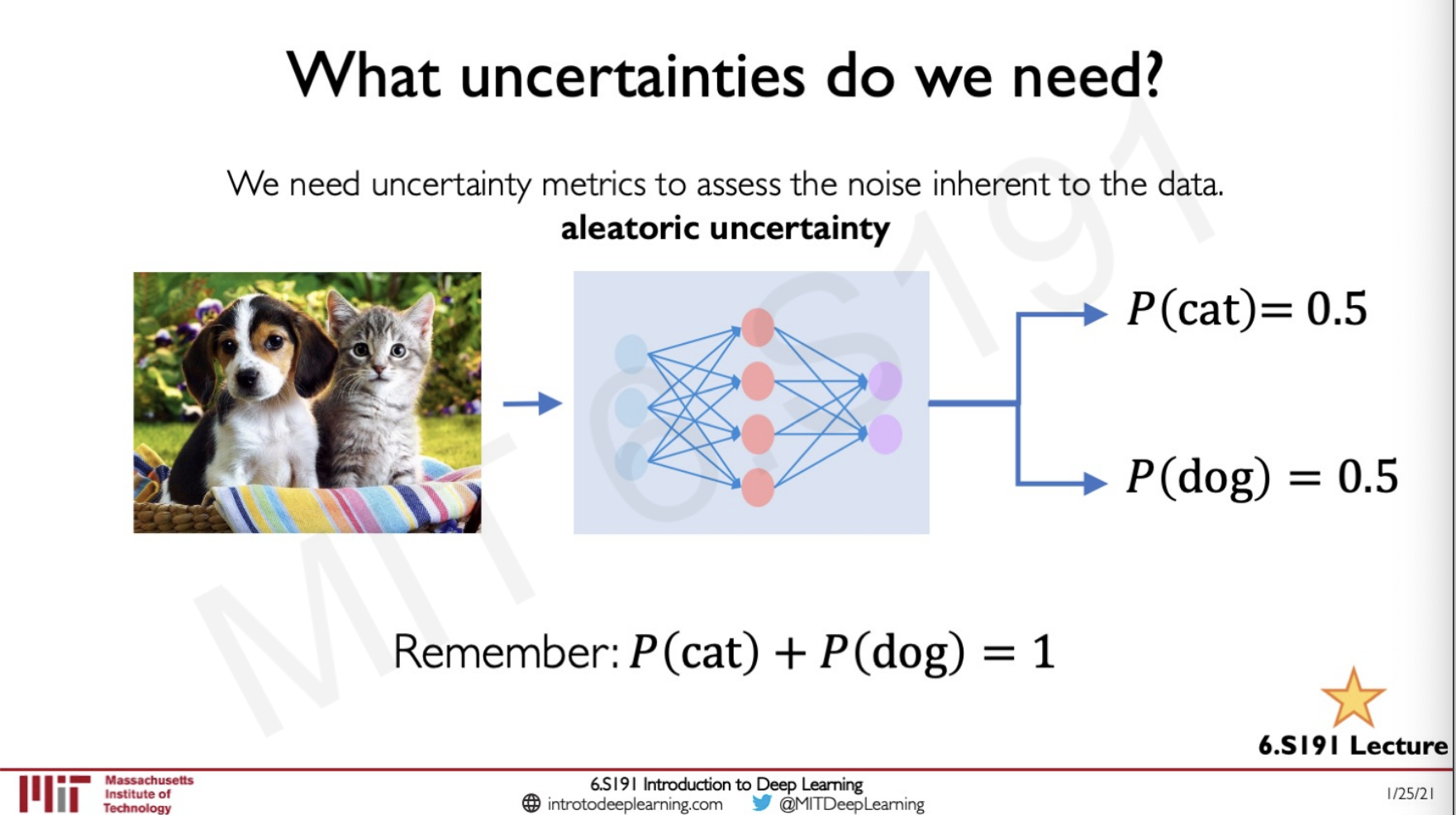When there is an uncertainty in the data this is called aleatoric uncertainty.

But now let us say that we pass in the model with an image of a horse now the model give out the prediction as follows.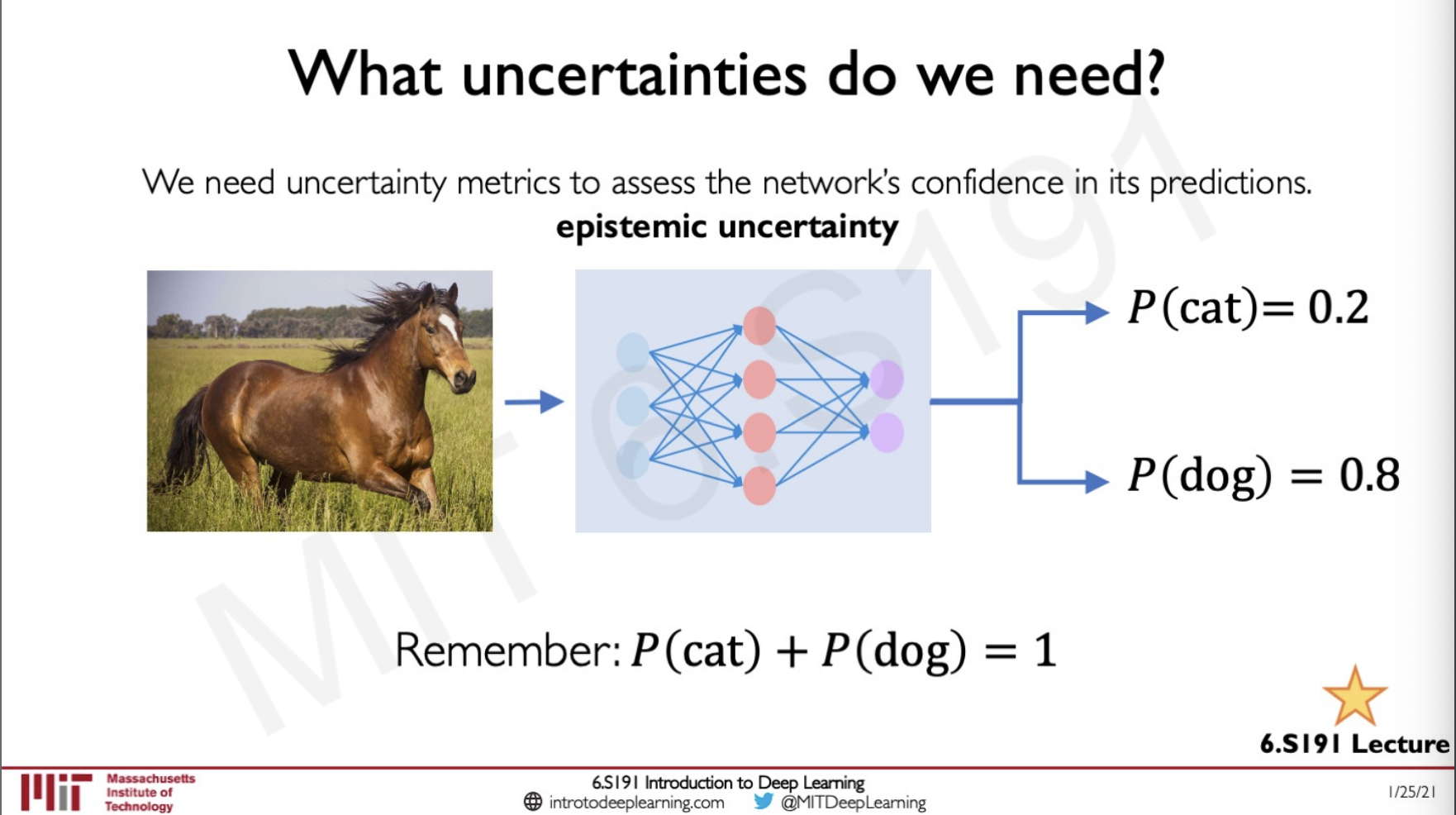Here we expect the model to give a wrong output but the model is very confident that the image is of a dog even though it is of a hourse.

This is an uncertainty in the model and is called an epistemic uncertainty.

There exist yet another method with which we can create uncertainty in a prediction this is by taking an image and adding some form of perturbations to the image so that it misclassifies.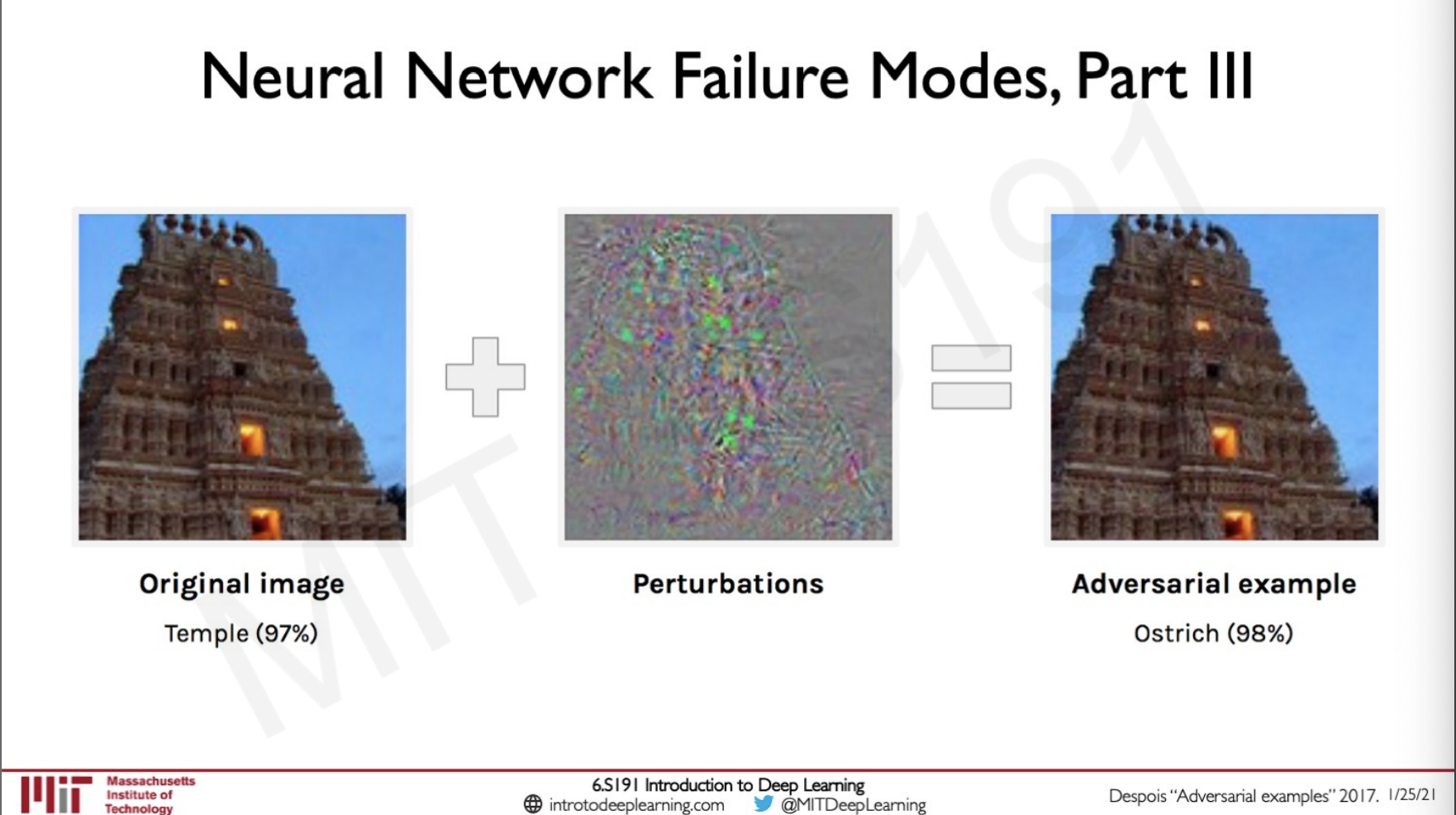Here we can see that the model miss-classifies the image of a temple as an ostrich which is a very bad uncertainty.

So what is this perturbation ?

To understand this we need to go back to how we train out neural network with gradient descent, we have our weights which are updated with a small change to decrease out loss.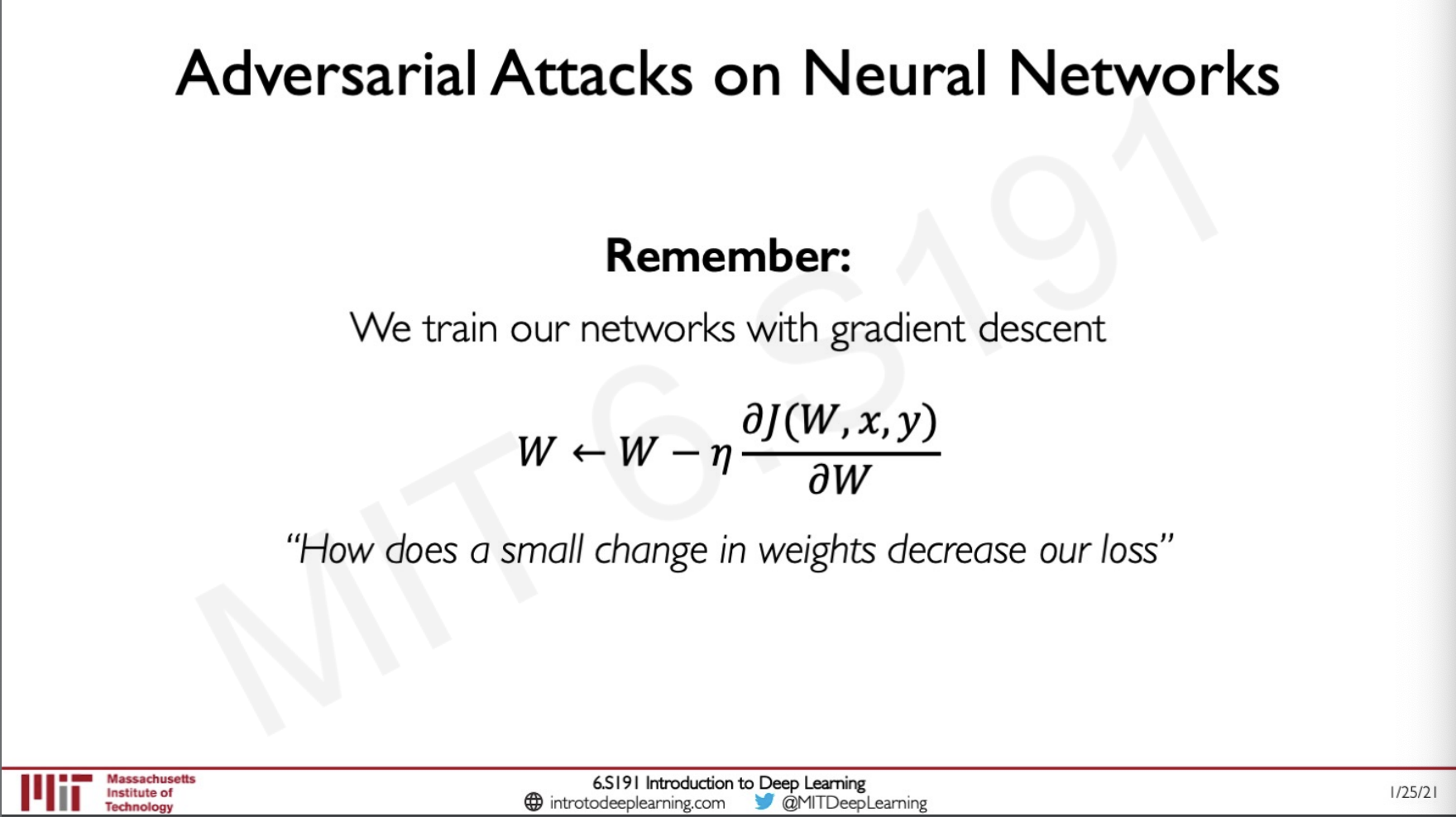Here we have keep the input image and label fixed and the changes happen on the weights.

In case of an adversarial attack we keep the weights and label fixed while changing the input image to increase the error so that the model misclassfies.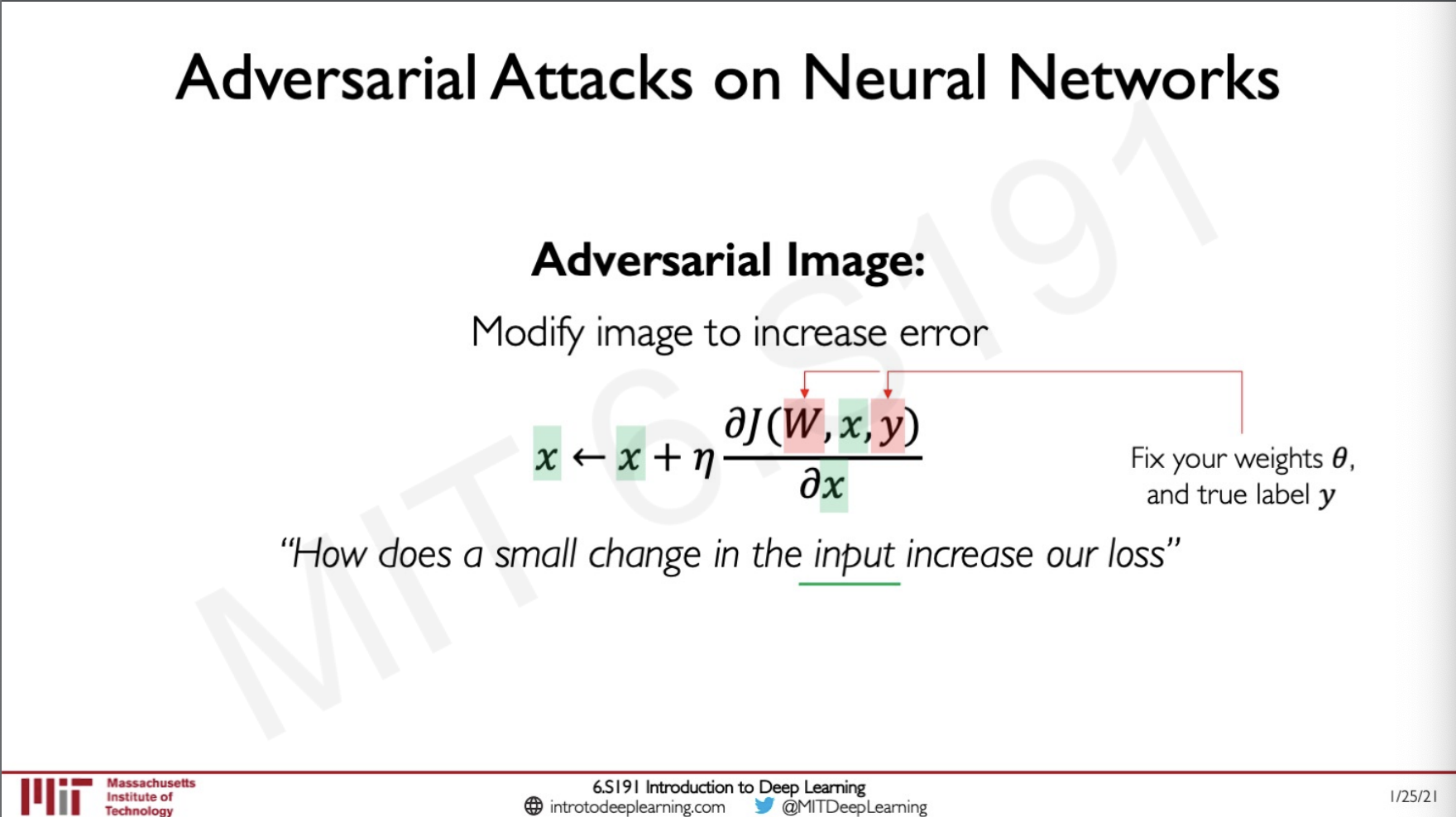https://arxiv.org/abs/1707.07397

Researchers at MIT have developed a GAN that can synthesise 2d images that can do an adversarial attack on a model.

They also went a step further to create a 3d model of a tortoise when shown to the model actually misclassify it as a rifle.

## Algorithmic Bias

As we have seen before the network is only as good as the data it is trained on, so there might be cases where the data from which the neural network is trained on may very representative this causes bias in the algorithm itself and would reflect in the implementation of the same.

## Neural Network Limitation

Some of the limitations of neural networks are

1. Very data hungry
2. Computationally intensive to train and deploy
3. Easily fooled by adversarial example
4. Can be subject to algorithmic bias
5. Poor at representing uncertanity
6. Uninterpretable black boxes, difficult to trust
7. Difficult to encode structure and prior knowledge during learning
8. Finicky to optimise non-convex, choice of architecture, learning parameters.
9. Often require expert knowledge to design, fine tune architectures

## New Frontiers in Deep Learning

### CNNs: Using Spatial Structure

we have already seen we can use a CNN to give a neural network the idea of spatial structure in an image, this is done in the following steps

1. Apply a set of weights to extract local features
2. Use multiple filters to extract different features
3. Spatially share parameters of each filter

Here the neural networks are built in such a way that it has two layers one for the feature learning stacked on top of the classification layer.

### Graph Convolutional Networks

As we know convolutional neural networks work by having a kernel that strides over and image capturing information of that image and then going to the next step.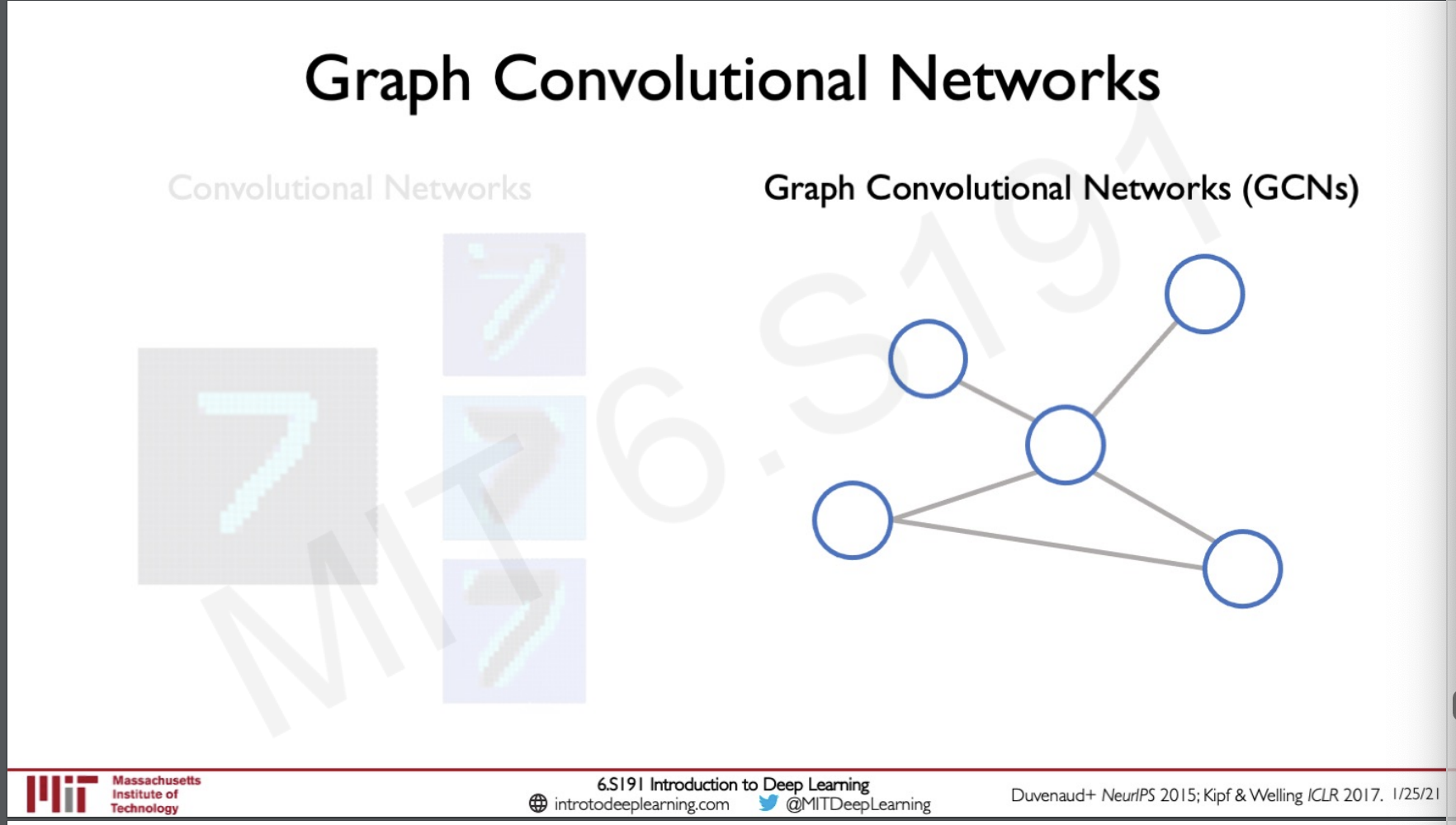Graph convolutional networks also work in a similar way they start at one node and the go to nearby nodes learning information from each one of them and then traverse to the next neighbour.

### Applications of Graph Neural Network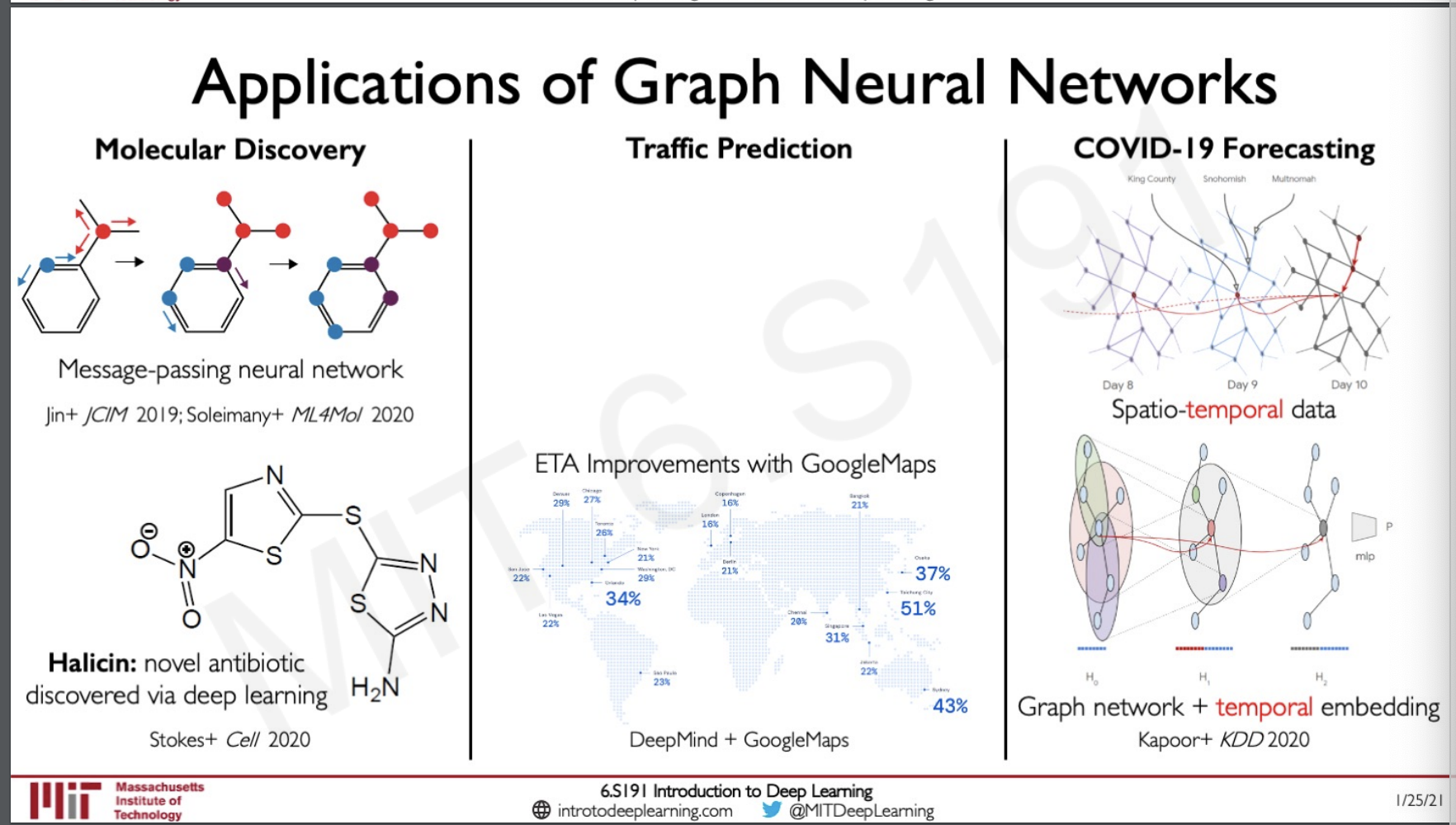Recently a variety of graph networks called Message-passing neural networks were used to learn data from molecules and create a novel antibiotic. Geographic data from Google Maps were used by DeepMind as Graphs and they were model-ed to predict traffic estimation in applications like google maps. Recently the data of the location of people along with temporal data of how the move around was fed into a neural network which has both graph and temporal component to predict the spread of covid-19.

### Learning from 3D data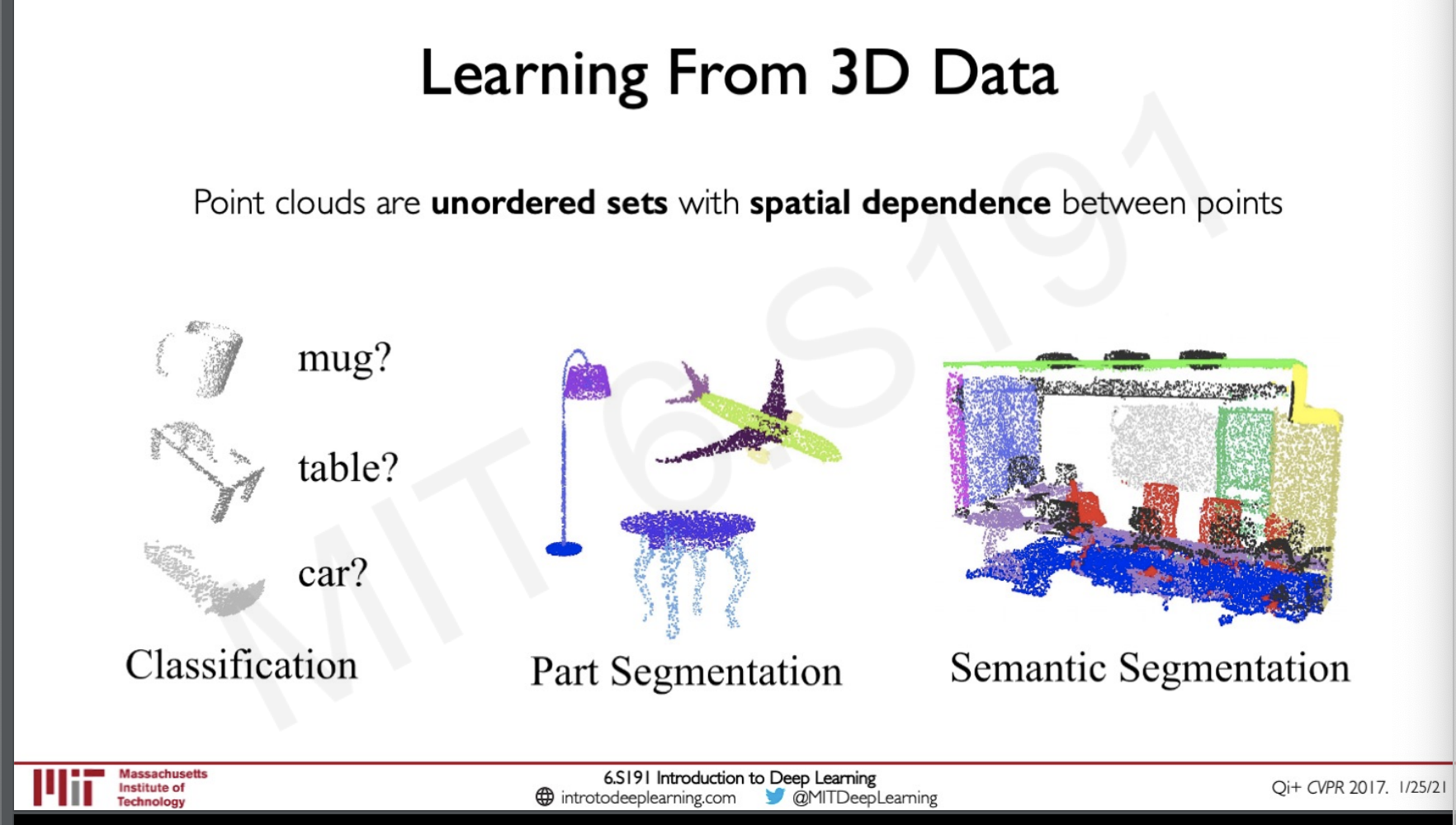Some of the task we have seen using 2d image data can also be performed in a 3d data by using point-clouds and graph convolutional networks, the points clouds are unordered sets with spatial dependencies between the points. These clouds are expanded and the points in them are locally connected and using a graph neural network patterns in these data are learned while spatial structure is also represented in the neural network.

## Automated Machine Learning

The problem with all the machine learning that we have learned so far is that a particular machine learning model is optimised with a particular task requiring lot of specialised engineers to do a lot of Research and Development to create new models to fit a task. What AutoML proposes is to create a machine learning model which learns the best model to use given a problem.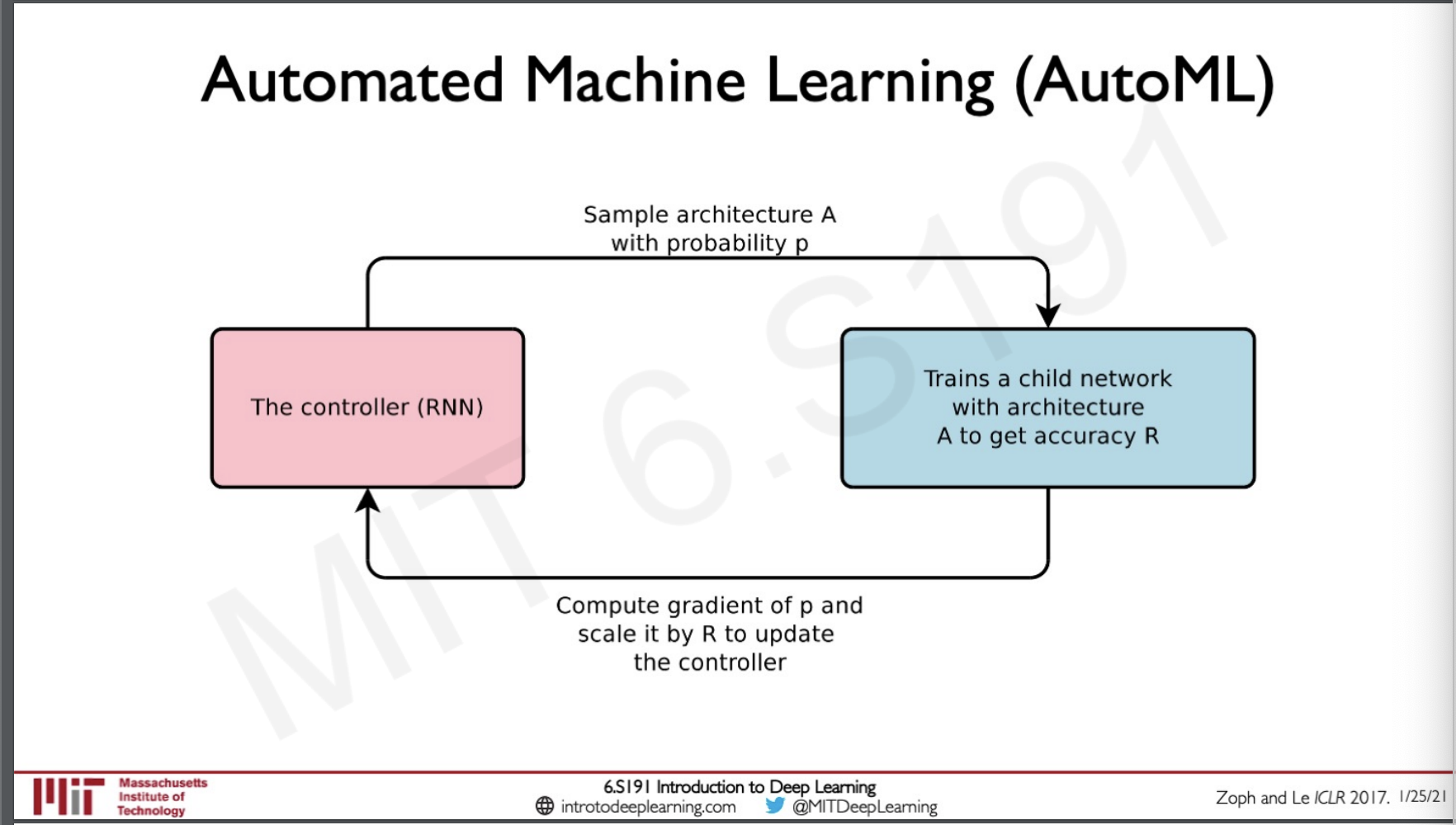This is the architecture of AutoML it has two components one is an RNN that generates the hyper-parameter to a proposed model and then the next component is a child network which is trained on these parameters to get the accuracy that can be used to update the controller.

### Model Controller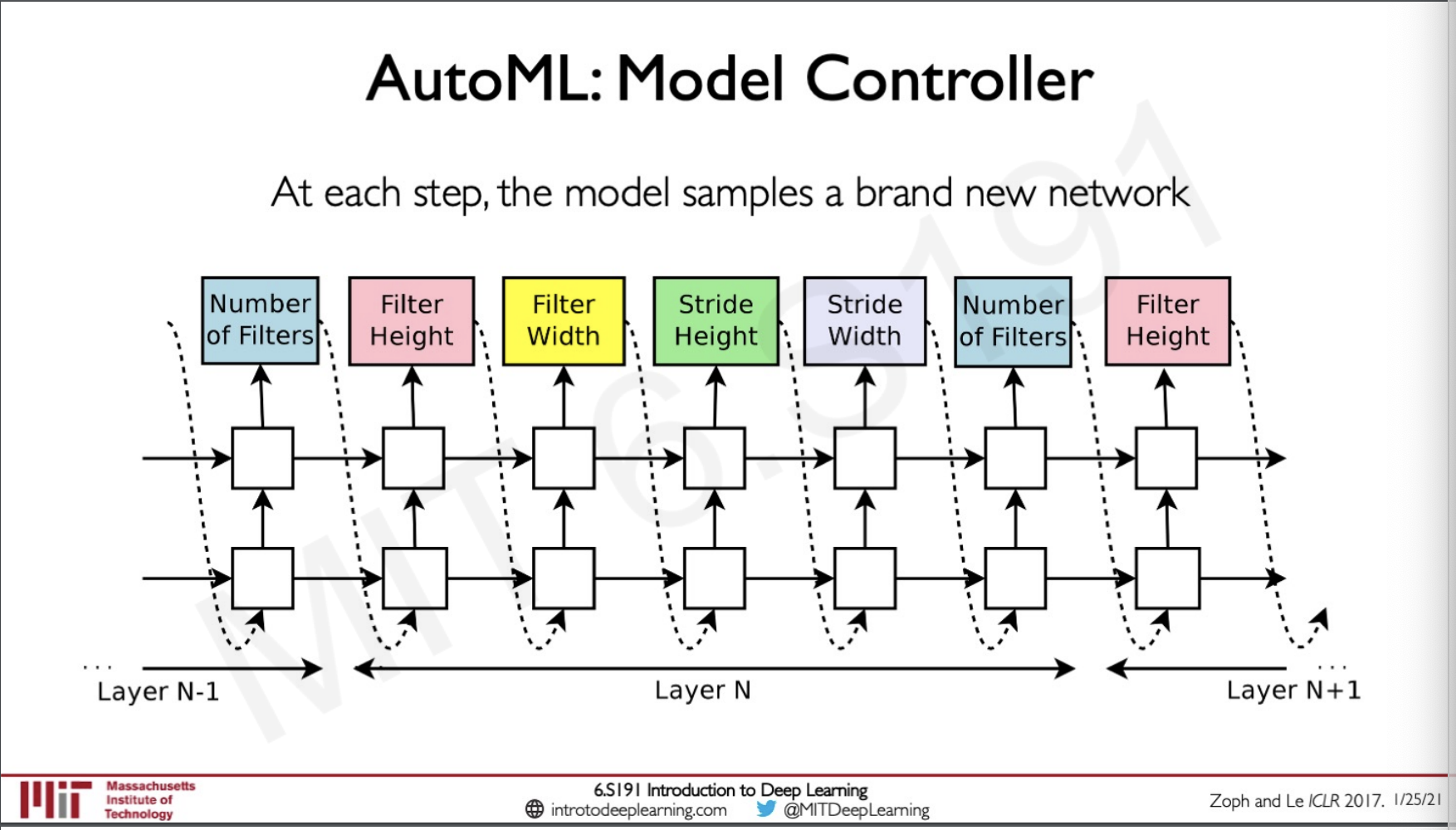The model controller basically samples a new network in every iteration, it is an RNN so as that the output represents a particular hyper-parameter and the same can be used as input to the next state to create the next hyper-parameter.

### The Child Network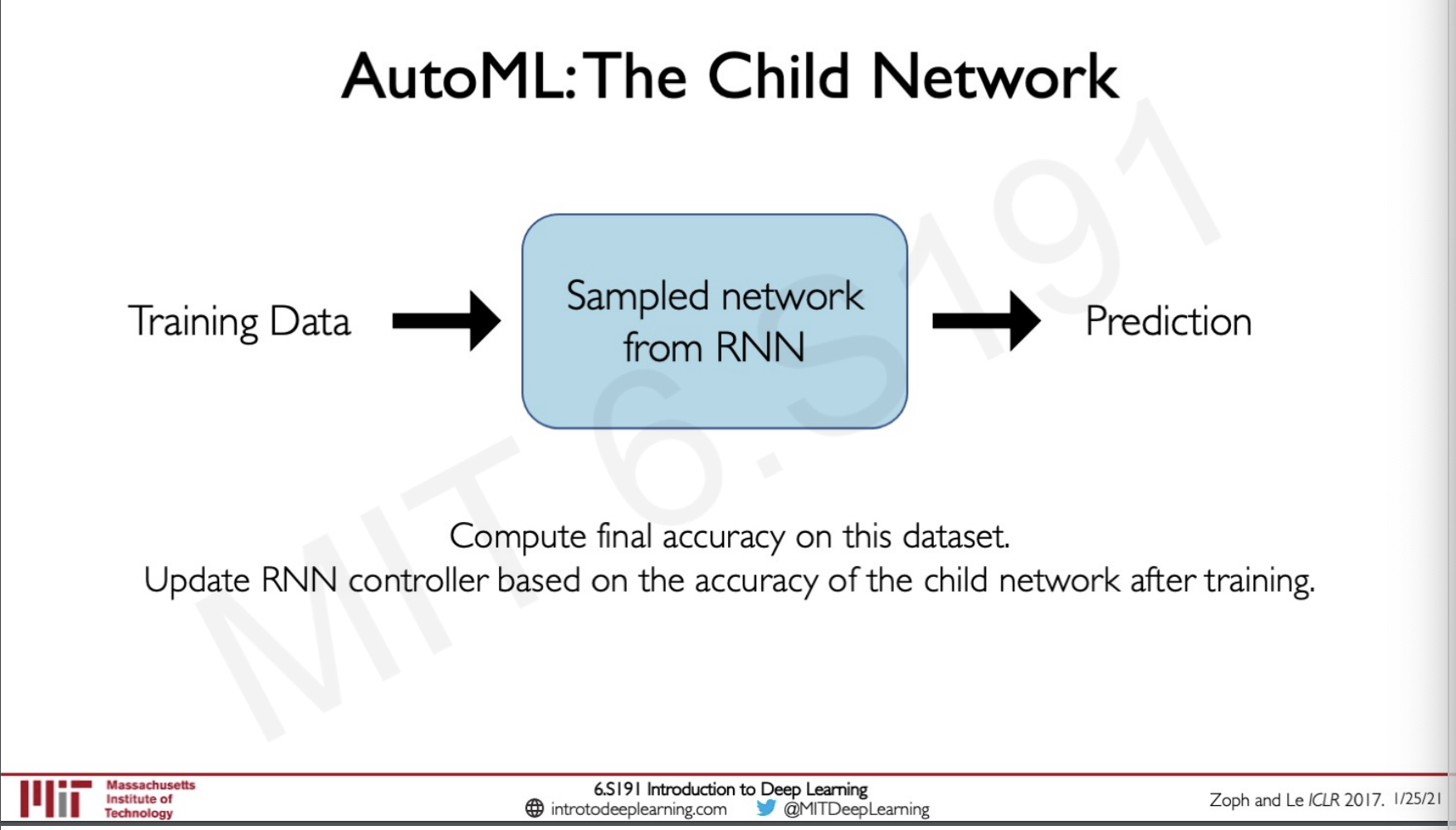The child network is basically a network that is build on top of the parameters given by the controller and the given training data, the output would be the predictions and we can use this to update our controller.

Recently a similar architecture was used for image recognition,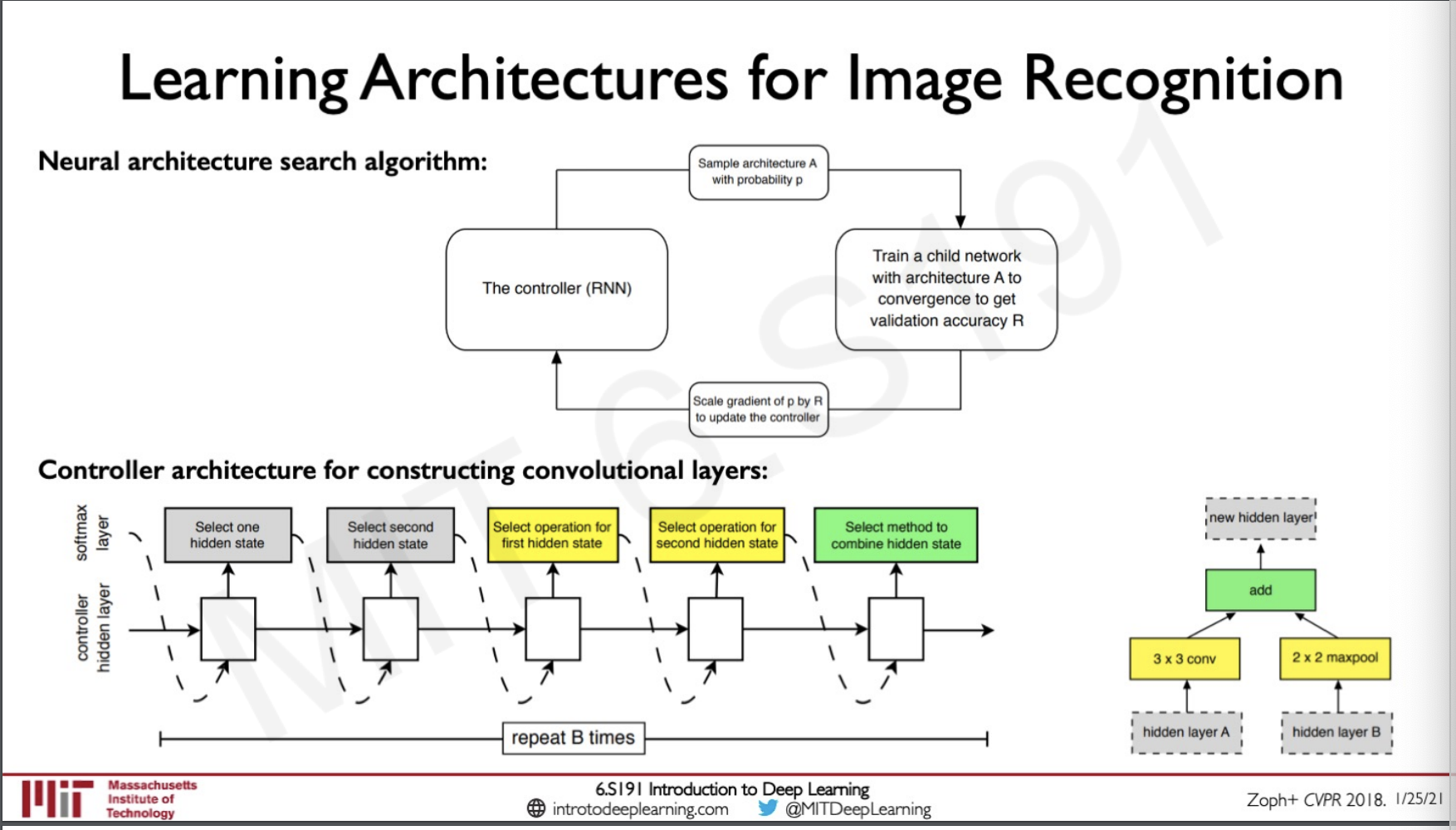After training this network the result was that the models trained by the AutoML system performed significantly compared to the system that was trained by experts.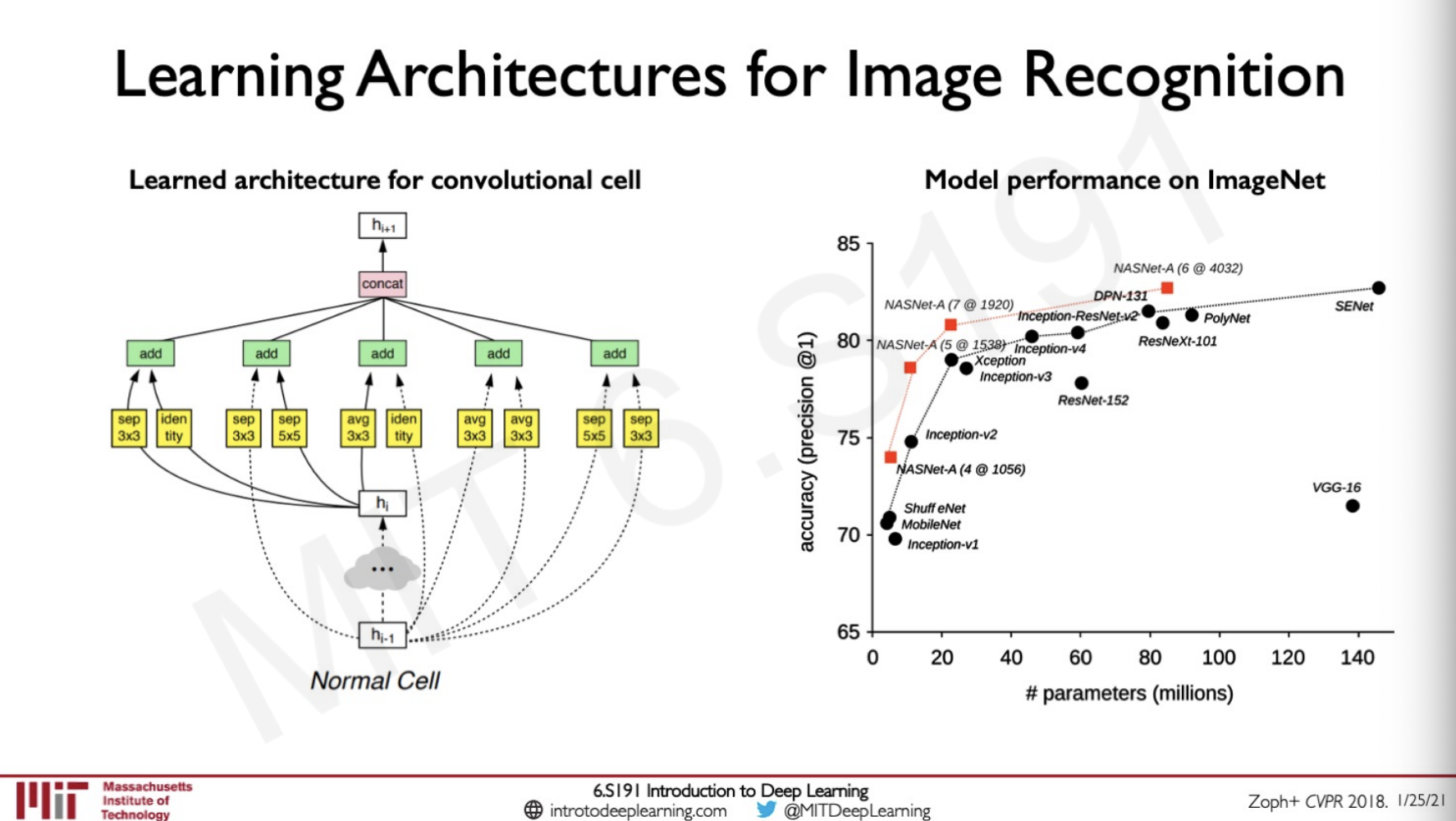So the idea is that here we are training neural network that are used to train neural networks.

This is also taken a step forward into creating something known as AutoAI.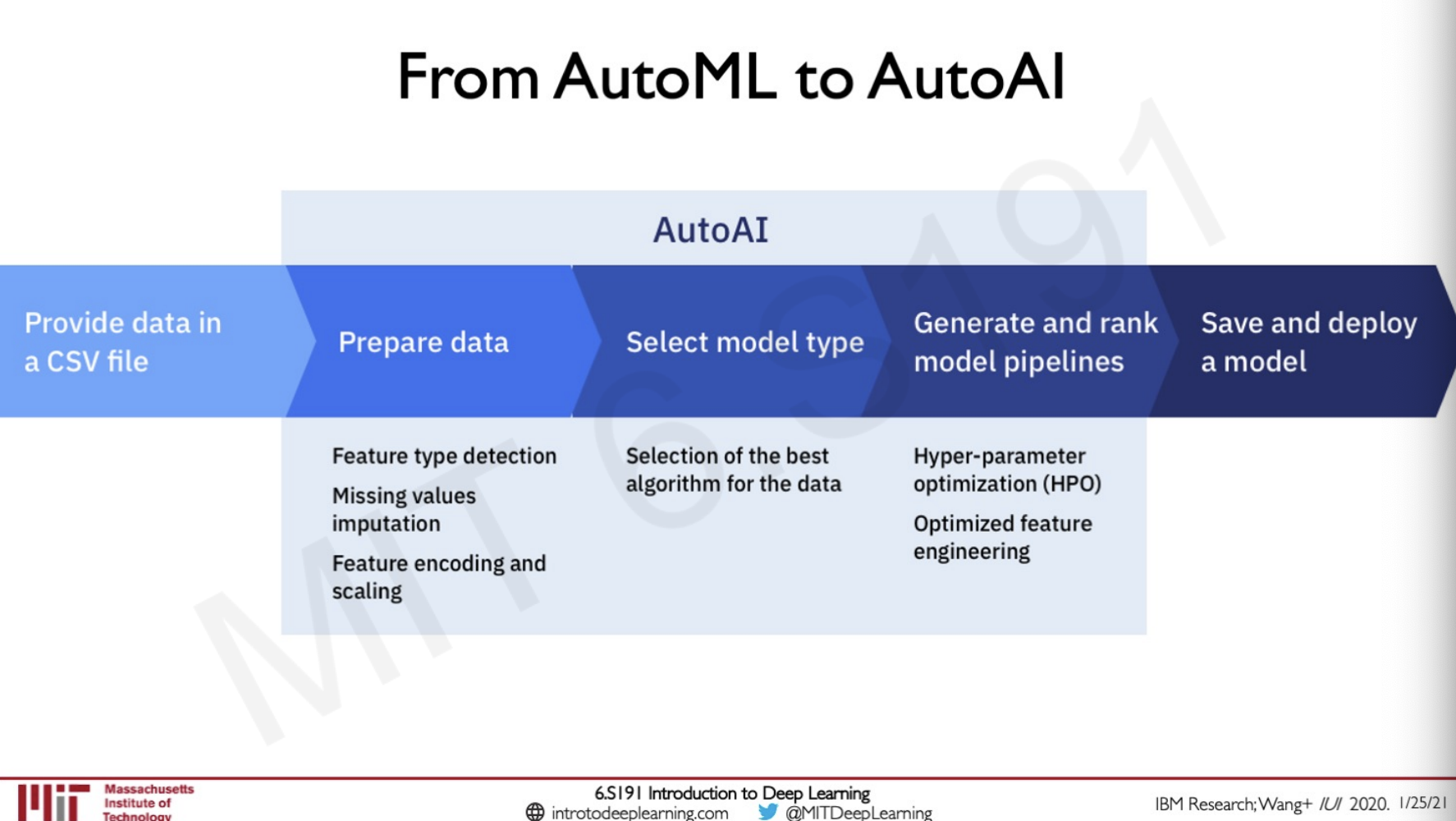This takes deep learning to a step forward and also makes it more accessible to the public given that they have enough and representative data to train models on.

## Sources

MIT introtodeeplearning : http://introtodeeplearning.com/

Slides on intro to deep learning by MIT :http://introtodeeplearning.com/slides/6S191_MIT_DeepLearning_L6.pdf

##### Subscribe to the newsletter

Get emails from me about machine learning, tech, statups and more.

- subscribers